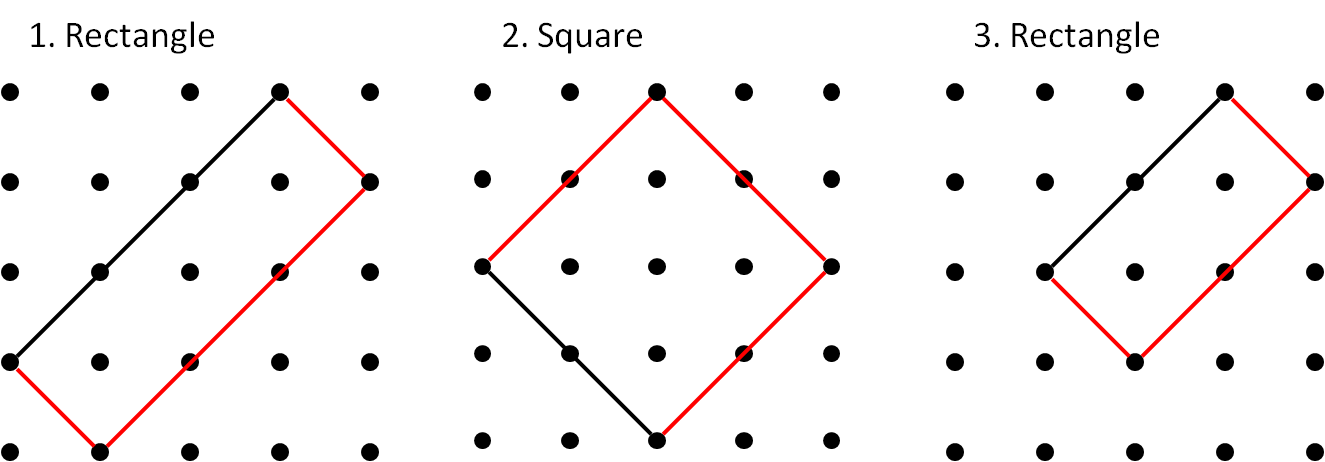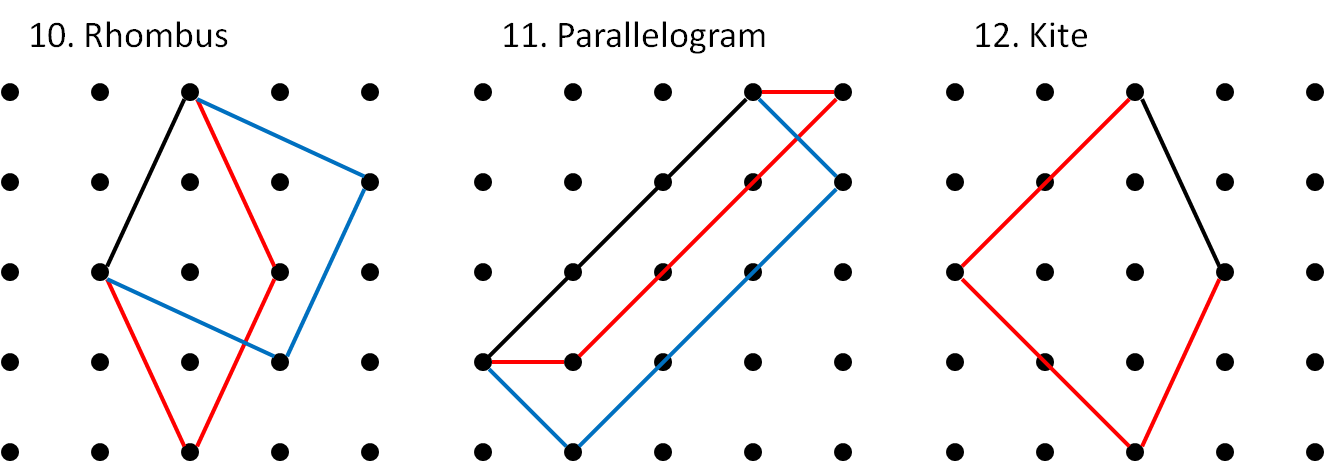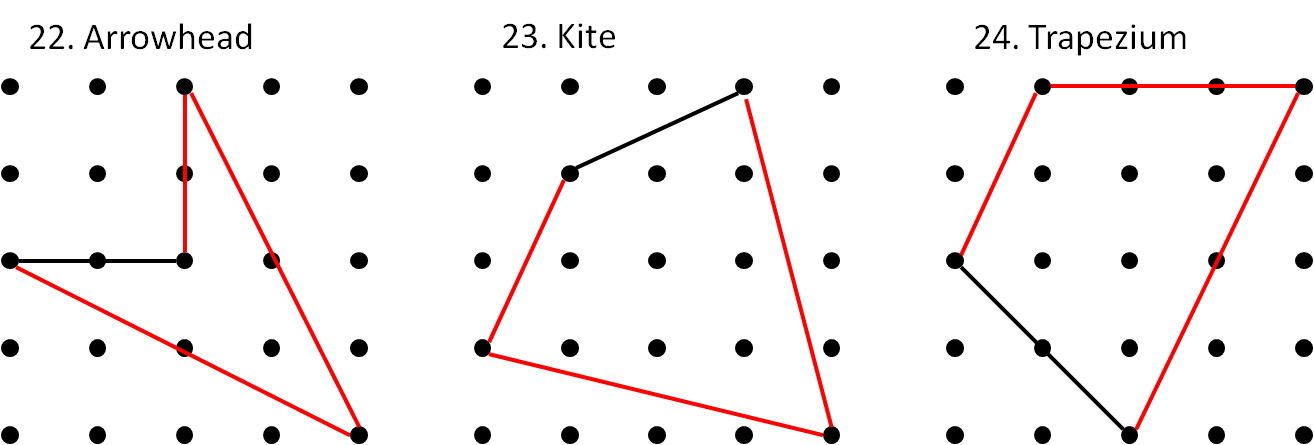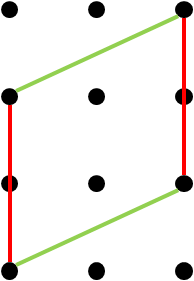#### You may also likeFour rods, two of length a and two of length b, are linked to form a kite. The linkage is moveable so that the angles change. What is the maximum area of the kite?### Making Rectangles, Making Squares

How many differently shaped rectangles can you build using these equilateral and isosceles triangles? Can you make a square?This gives a short summary of the properties and theorems of cyclic quadrilaterals and links to some practical examples to be found elsewhere on the site.

##### Age 11 to 14Challenge Level

Solutions to this problem were received from Emma, Rebekah and Charlotte, all of Wycombe High School, Julian, from the British School Manila in the Philippines, Ian, from Canberra Grammar School, Australia, and Zach. Thank you and well done to them!

The solution presented below is a combination of all of their solutions.

You will have made a decision about whether allowed special cases in your solutions, such as allowing a square when a rectangle is asked for. Take a look at the Getting Started page if you want to find out more about special cases.

In the solutions below, the red shapes exclude special cases. Where there is a special case that is larger, that shape is given in blue.One common mistake was to make a shape where the sides were not quite of the same length. For example, consider the shape below:This was often claimed to be a rhombus, but the red and green sides are not of the same length. The red sides are of length $2$, whereas the green sides are of length $\sqrt{5}$. If you want to understand why these are of different lengths, either ask your teacher about Pythagoras' theorem, or take a look at Tilted Squares.